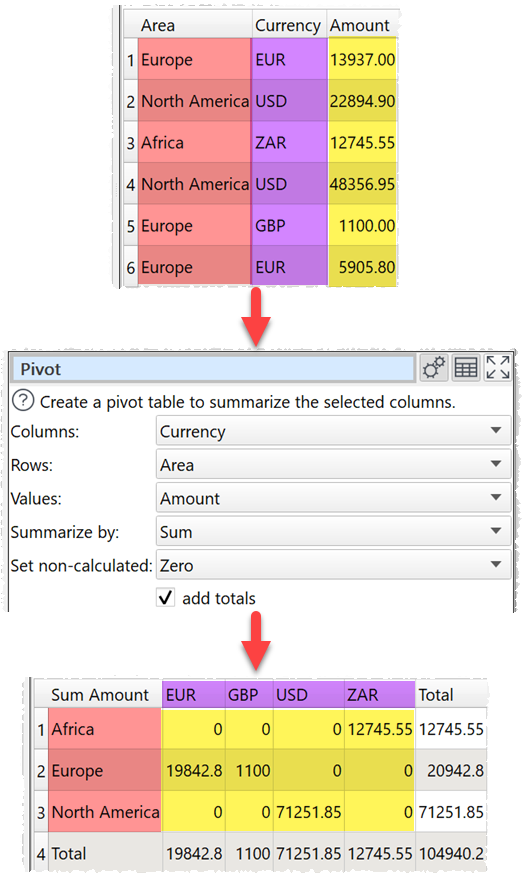﻿ Reference > Transforms > Pivot

# Pivot

Navigation:  Reference > Transforms >

# Pivot

## Description

Creates a pivot table to summarise values for one or two columns.

## Example

Pivot to sum 'Amount' by 'Area' and 'Currency':One.

## Options

Set Column to the column values you want to use as columns in your pivot table.

Set Rows to the column values you want to use as rows in your pivot table.

Set Values to the column you wish to summarize.

Set Summarize by to how you wish to summarize the values:

oSum show the sum of the values. Non-numeric and empty values are ignored.

oMinimum shows the smallest value. Non-numeric and empty values are ignored.

oMaximum shows the largest value. Non-numeric and empty values are ignored.

oAverage shows the arithmetic mean of the values. Non-numeric and empty values are ignored.

oMedian shows the median of numeric values in the column. Non-numeric and empty values are ignored.

oMode shows the mode of numeric values in the column. Non-numeric and empty values are ignored.

oStandard deviation is the sample standard deviation (equivalent to Excel function stddev.s). Non-numeric and empty values are ignored.

oCount shows the number of values. Empty values are ignore (a value that contains whitespace is not considered empty).

Set Set non-calculated depending on how you want to set cells not calculated by the pivot.

Check add totals to add row and/or column totals.

## Notes

Mode may not work as expected with non-integer values, due to precision issues. You can fix this by using a Num Format transform first.

Use Num Format to change the precision of the results.